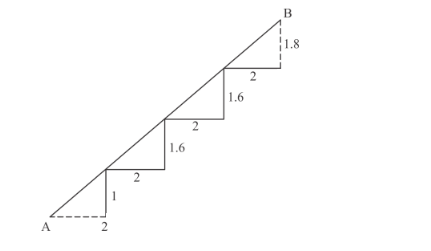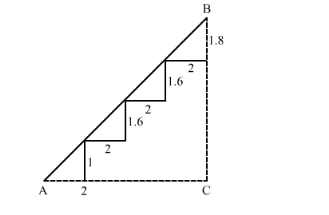# There is a staircase as shown in the given figure,

Question:

There is a staircase as shown in the given figure, connecting points A and B. Measurements of steps are marked in the figure. Find the straight line distance between A and B.Solution:

We are given the following figure with the related informationIn the above figure complete the triangle ABC with right angled at C

So

AC = 2 + 2 + 2 + 2 = 8 and

BC = 1 + 1.6 + 1.6 + 1.8 = 6

Using Pythagoras theorem for triangle ABC to find

$A B^{2}=A C^{2}+B C^{2}$

$=8^{2}+6^{2}$

$=100$

$\Rightarrow A B=10$

Hence the distance between $A$ and $B$ is